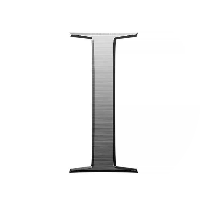# Number Theory and Cryptography

This quiz contains multiple-choice problems on prime numbers, quadratic residue, LCM, HCF, base conversion, exponent rules, number complement, number theory applications, modular exponentiation, cyphers, cryptography encryption and decryption.

Start Quiz

All prime numbers are odd. True or false?

True

False

3 is the smallest prime number possible. True or false?

True

False

How many prime numbers exist between 1 to 20?

5

6

7

None of the above

There are a finite number of prime numbers. True or false?

True

False

The sum of two distinct prime numbers is

A prime number

A composite number

Either prime or composite

None of the above

The difference between two distinct prime numbers is

Odd and prime

Even and composite

All of the above

None of the above

If a and b are two distinct prime numbers, then the highest common factor of a and b is

2

0

1

ab

If a, b, c and d are distinct prime numbers whose least common factor is the smallest prime, then a * b * c * d is a/an

Odd number

Even number

Prime number

None of the above

The one’s complement in binary is defined as

Flipping each binary bit

Adding one to the binary number

Flipping only the bits having zero in it

None of the above

What is the one’s complement of the number 1010110?

1111111

0101001

1100110

None of the above

If the one’s complement of a number x is y, then the one’s complement of y is

y

x

x + y

None of the above

The nine’s complement of a number is formed by

Replacing each digit by 9 minus that digit

Replacing each digit by 1 plus that digit

Replacing each digit by 8 minus that digit

None of the above

What is the nine’s complement of 23456?

87654

76543

12345

None of the above

The number of factors that prime numbers have is

2

3

Depends on the prime number

None of the above

What is the number 1?

A prime number

A composite number

Neither prime nor composite

None of the above

Quiz/Test Summary
Title: Number Theory and Cryptography
Questions: 15
Contributed by: